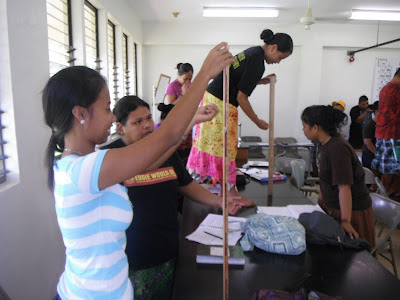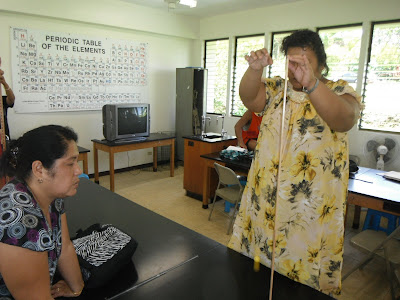### Ball bounce height lab

Laboratory 14 is intended as a form of practical examination. The students investigate a physically simply system and then have to determine the mathematical model underlying that system.Laurie and Tommy, their super ball in mid-flight

Summer 2012 the students explored the bounce number versus bounce height. The students then have to write up their analysis of the system based on their data. Their laboratory report is marked both for content and syntax.Tommy notes the bounce height

The objective is to determine when given a system that they have never worked with before, the students can work their way through to a mathematical analysis. This system has a twist in the mathematical model. The students are more familiar with a linear analysis, and points are given for running with the familiar. Full credit, however, requires following the data wherever it leads mathematically. During the term the students have seen quadratic and square root mathematical models, this one pushes into other less familiar terrain.Kramwel and JohnsonGasma and MelydaArthy and ArnaldJacky records data while May-vy tracks the bounce heightBeverleen dropsRosalina "Miuky" drops the ball while Judy watches for the bounceJudy Kostka